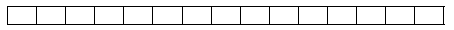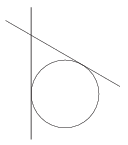Mathematical and Physical Journal
for High Schools
Issued by the MATFUND Foundation
 Already signed up? New to KöMaL?

# KöMaL Problems in Mathematics, November 2015

Please read the rules of the competition.

Show/hide problems of signs:## Problems with sign 'K'

Deadline expired on December 10, 2015.

K. 475. In the fields in the table (see the figure), enter the integers 1 to 15 so that the sum of the two numbers in any two adjacent field is a perfect square.(6 pont)

solution, statistics

K. 476. Find all positive integers for which the number obtained by rounding to the nearest thousands is twice the number obtained by rounding to the nearest hundred. (As a rule, numbers ending in 5, 50, 500, $\displaystyle \dots\;$ round upwards.)

(6 pont)

solution, statistics

K. 477. Johnny is trying to learn how to eat with chopsticks. To practice, he needs to lift a ball of diameter 4 cm with the chopsticks, as shown in the diagram. (A ball can be lifted if its centre lies in the plane of the two sticks.) The two chopsticks in Johnny's hand enclose an angle of 60 degrees. Find the distance of the crossing point of the two sticks from the closest point of the ball during this operation.(6 pont)

solution, statistics

K. 478. Farmer Thomas wanted to buy 4 metres of chain that costs 210 forints (HUF, Hungarian currency) a metre. The shop assistant tried to talk him into buying all the 10 metres they have in stock, but Farmer Thomas insisted on buying 4 metres only. However, he noticed that the assistant, on purpose, made a mistake in measuring the 4 metres, and cut off a shorter piece. Therefore he decided to ask for the other piece instead, which the assistant had to sell him for the price of 6 metres in order to avoid being caught cheating. Had he not noticed the cheating, it would have cost Farmer Thomas 14/9 as much to buy a metre of chain as it actually cost with this clever manoeuvre. How many metres of chain did Thomas get?

(6 pont)

solution, statistics

K. 479. In the expression $\displaystyle \big({(-a^{-b})}^{-c}\big)^{-d}$ the numbers 1, 2, 3, 4 are substituted for $\displaystyle a$, $\displaystyle b$, $\displaystyle c$, $\displaystyle d$ in some order. In which case will the value of the expression be a maximum, and in which case will it be a minimum?

(6 pont)

solution, statistics

K. 480. In the following addition, the five letters stand for the five odd digits in some order: $\displaystyle a+\overline{bb} +\overline{ccc\vphantom{b}} +\overline{dddd} +\overline{eeeee\vphantom{b}}$. Find the sum of all five-digit numbers that can be represented in this form.

(6 pont)

solution, statistics## Problems with sign 'C'

Deadline expired on December 10, 2015.

C. 1315. In a chocolate factory, chocolate mass is poured into molds to make 100-gram bars. Owing to a malfunction of machinery, one out of 45 bars breaks in the process. A quality control inspector spots these broken bars before they would get wrapped, and returns them to the molten chocolate mass. However, the inspector misses one out of 21 broken bars and lets them go on to the wrapping machine. Out of 10 tonnes of chocolate mass, how many broken bars of chocolate will get to the market?

(5 pont)

solution, statistics

C. 1316. In a classroom there are 18 seats altogether, forming 3 columns and 6 rows. 10 girls and 7 boys sit down in the classroom. How many different seating arrangements are possible if no row or column may consist of all boys or all girls?

(5 pont)

solution, statistics

C. 1317. The interior angles lying at vertices $\displaystyle A$, $\displaystyle B$, $\displaystyle C$ and $\displaystyle D$ of a pentagon $\displaystyle ABCDE$ are $\displaystyle 90^\circ$, $\displaystyle 60^\circ$, $\displaystyle 150^\circ$ and $\displaystyle 150^\circ$, respectively. Furthermore $\displaystyle AB=2BC=\frac 43 AD$. Prove that the line segment joining the intersection of lines $\displaystyle AE$ and $\displaystyle CD$ to the intersection of lines $\displaystyle AD$ and $\displaystyle BC$ is parallel to $\displaystyle AB$.

(5 pont)

solution, statistics

C. 1318. The number 518 has an interesting property. Consider the six three-digit numbers obtained with the different permutations of the digits of 518. The mean of these numbers is equal to 518. Find all three-digit numbers of different digits with this property.

(5 pont)

solution, statistics

C. 1319. The midpoints of the sides of a quadrilateral form a square. The area of the quadrilateral is 50, and two opposite sides are 5 and $\displaystyle \sqrt{85}\,$. How long are the other two sides?

(5 pont)

solution, statistics

C. 1320. Solve the following equation on the set of real numbers: $\displaystyle 4x^{2}y^{2}+z^{4}+\sqrt{3x^{2}y-6x^{2}}+16=7z^{2}+4xyz$.

(5 pont)

solution, statistics

C. 1321. How many different simple graphs of 6 vertices and 5 edges are there?

(5 pont)

solution, statistics## Problems with sign 'B'

Deadline expired on December 10, 2015.

B. 4741. How many different triangles with an axis of symmetry are there in which one side is twice as long as one of the altitudes? Similar triangles are not considered different.

(3 pont)

solution, statistics

B. 4742. Show that it is possible to label the edges of a complete graph of $\displaystyle n\ge 3$ vertices with 1, 2 or 3, so that the product of the labels of the edges be different at each vertex.

(4 pont)

solution, statistics

B. 4743. The inscribed circle of triangle $\displaystyle ABC$ touches sides $\displaystyle BC$, $\displaystyle AC$ and $\displaystyle AB$ at points $\displaystyle A_1$, $\displaystyle B_1$ and $\displaystyle C_1$, respectively. Let the orthocentres of triangles $\displaystyle AC_1B_1$, $\displaystyle BA_1C_1$ and $\displaystyle CB_1A_1$ be $\displaystyle M_A$, $\displaystyle M_B$ and $\displaystyle M_C$, respectively. Show that triangle $\displaystyle A_1B_1C_1$ is congruent to triangle $\displaystyle M_AM_BM_C$.

Proposed by Sz. Miklós, Herceghalom

(4 pont)

solution, statistics

B. 4744. Let $\displaystyle n$ be a non-negative integer. Determine the exponent of 7 in the prime factor representation of $\displaystyle 3^{7^n} + 4^{7^n}$.

Proposed by K. Williams, Szeged

(5 pont)

solution, statistics

B. 4745. Let $\displaystyle n$ be a positive integer. Solve the equation $\displaystyle \frac{1}{\sin^{2n} x} + \frac{1}{\cos^{2n} x} = 2^{n+1}$.

Proposed by L. Longáver, Szatmárnémeti

(4 pont)

solution, statistics

B. 4746. The inscribed circle of triangle $\displaystyle ABC$ touches sides $\displaystyle BC$, $\displaystyle AC$ and $\displaystyle AB$ at points $\displaystyle A_1$, $\displaystyle B_1$ and $\displaystyle C_1$, respectively. The other intersection of line segment $\displaystyle AA_1$ with the inscribed circle is $\displaystyle Q$. The line through point $\displaystyle A$ parallel to $\displaystyle BC$ intersects the lines $\displaystyle A_1 C_1$ and $\displaystyle A_1 B_1$ at points $\displaystyle P$ and $\displaystyle R$. Prove that $\displaystyle PQR\sphericalangle =B_1 QC_1\sphericalangle$.

(Kvant)

(5 pont)

solution, statistics

B. 4747. In a certain lottery game, 6 numbers are drawn every week, out of the numbers 1 to 45. The draw of the first week of this year produced surprising results, since five consecutive numbers appeared. The numbers drawn were 37, 38, 39, 40, 41, 45. The news spread fast in the press. The question arises whether the excitement was justified: are these numbers so special? Let a number sequence be called perfect if it consists of six consecutive numbers, and nearly perfect if exactly five numbers out of the six are consecutive. How many perfect and nearly perfect combinations are there? Considering that the lottery game has been played for 26 years, and there have been 1227 weekly draws so far, what is the probability that during a time interval of this length at least one perfect or nearly perfect sequence of numbers is drawn?

Proposed by M. E. Gáspár, Budapest

(3 pont)

solution, statistics

B. 4748. A triangle $\displaystyle \mathcal H$ is rotated about a line lying in its plane but not intersecting it. Show that the volume of the resulting solid equals the product of the area of $\displaystyle \mathcal H$ and the perimeter of the circle described by the centroid of $\displaystyle \mathcal H$ during the rotation.

(5 pont)

solution, statistics

B. 4749. The feet of the altitudes drawn from vertices $\displaystyle B$ and $\displaystyle C$ of an acute-angled triangle $\displaystyle ABC$ on the sides $\displaystyle AC$ and $\displaystyle AB$ are $\displaystyle D$ and $\displaystyle E$, respectively. The midpoint of side $\displaystyle BC$ is $\displaystyle F$. The intersection of line segments $\displaystyle AF$ and $\displaystyle DE$ is $\displaystyle M$, and the orthogonal projection of point $\displaystyle M$ onto the line segment $\displaystyle BC$ is $\displaystyle N$. Prove that line segment $\displaystyle AN$ bisects line segment $\displaystyle DE$.

Proposed by B. Bíró, Eger - In memoriam Attila Kálmán

(6 pont)

solution, statistics## Problems with sign 'A'

Deadline expired on December 10, 2015.

A. 653. Let $\displaystyle n\ge2$ be an integer. Prove that there exist integers $\displaystyle a_1,\dots,a_{n-1}$ such that $\displaystyle a_1 \arctg 1 + a_2 \arctg 2 +\ldots+ a_{n-1}\arctg(n-1) = \arctg n$ if and only if $\displaystyle n^2+1$ divides $\displaystyle (1^2+1)(2^2+1)\ldots\big((n-1)^2+1\big)$.

Based on a problem of IMC 2015, Blagoevgrad

(5 pont)

solution, statistics

A. 654. Let $\displaystyle p(x)$ be a polynomial of degree at most $\displaystyle n$ such that $\displaystyle \big|p(x)\big|\le\frac{1}{\sqrt{x}}$ for $\displaystyle 0<x\le 1$. Prove that $\displaystyle \big|p(0)\big|\le 2n+1$.

(5 pont)

solution, statistics

A. 655. Two circles, $\displaystyle k_1$ and $\displaystyle k_2$ meet at points $\displaystyle A$ and $\displaystyle B$. Points $\displaystyle C$ and $\displaystyle D$ lie on $\displaystyle k_1$, while points $\displaystyle E$ and $\displaystyle F$ lie on circle $\displaystyle k_2$ in such a way that $\displaystyle A$, $\displaystyle C$, $\displaystyle E$ are collinear and $\displaystyle B$, $\displaystyle D$, $\displaystyle F$ are collinear, too. Points $\displaystyle G$ and $\displaystyle H$ are other two points on lines $\displaystyle ACE$ and $\displaystyle BDF$, respectively. The line $\displaystyle CH$ meets $\displaystyle FG$ and $\displaystyle k_1$ the second time at $\displaystyle I$ and $\displaystyle J$, respectively. The line $\displaystyle DG$ meets $\displaystyle EH$ and $\displaystyle k_1$ the second time at $\displaystyle K$ and $\displaystyle L$, respectively. Circle $\displaystyle k_2$ meets the lines $\displaystyle EHK$ and $\displaystyle FGI$ the second time at $\displaystyle M$ and $\displaystyle N$, respectively. The points $\displaystyle A,B,C,\ldots,N$ are distinct. Show that $\displaystyle I$, $\displaystyle J$, $\displaystyle K$, $\displaystyle L$, $\displaystyle M$ and $\displaystyle N$ are either concyclic or collinear.

(5 pont)

solution, statistics

### Upload your solutions above or send them to the following address:

KöMaL Szerkesztőség (KöMaL feladatok),
Budapest 112, Pf. 32. 1518, Hungary